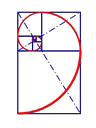## [問題]求a,b,c### [問題]求a,b,c

a,b,c∈Na+3b=2c,2a^2+c^2=3b^2，又[a,b,c]=300，求a,b,c之值。tangpakchiua+3b=2c=>a+b=2(c-b),a=2c-3b
2a^2+c^2=3b^2=>2(a+b)(a-b)=(b+c)(b-c)
∵b≠c

15a=3c=5b...(a:b:c=1:3:5)
=>abc=15a^3,(a,b,c)=a

[a,b,c]=300
=abc/(a,b,c)^2=15a
a=20
b=60
c=100

~就讓一切隨風~lcflcflcf